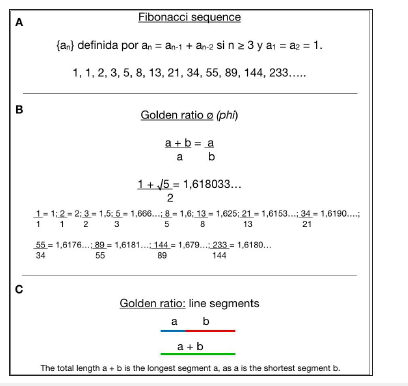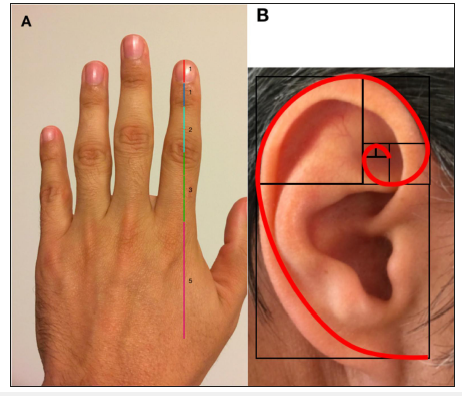• Submissions# Relationship between the Biliary Tree and the Fibonacci Sequence

Carlos Federico Davrieux1* and Juan Agustín Davrieux2

1Argentina

2Department of Economic Sciences and Statistics, Argentina

*Corresponding author:Carlos Federico Davrieux, Argentina

Submission: April 18, 2019;Published: May 29, 2019ISSN 2637-7632
Volume3 Issue2

#### Abstract

Purpose: Mathematics has been one of the sciences that have been most applied in various fields. One of these examples is the “Fibonacci series”, related to the golden proportion. The Fibonacci numbers can be found in geometry, botany, ancient architecture, animal kingdom, and the human body, among other areas. The distribution of human anatomy of the biliary tree could follow the Fibonacci sequence. The aim is to determine the relationship between the anatomical distribution of the human biliary tree and the mathematical Fibonacci´s sequence.

Methods: Observational descriptive study that sought to relate the Fibonacci series with the anatomy of the biliary tree. A search and bibliographic analysis of the relation of the Fibonacci´s sequence to medicine was carried out.

Result: From the physiological point of view, the liver can be divided into eight segments, four right and three left, plus segment I. We relate the anatomical distribution of the biliary tree with the Fibonacci sequence.

Conclusion: It is possible to associate the anatomical distribution of the human biliary tree with the mathematical Fibonacci sequence. This could help the compression of the anatomical variables of the biliary tract and avoid surgical lesions of the same.

Keywords: Fibonacci series; Gold ratio; Anatomy; Biliary tree; Liver

#### Introduction

Throughout the years, mathematics has been one of the sciences that have been most applied in various fields. Several scientists and mathematicians have cooperated in the advancement of this science with the development of theorems, theories, and laws. These concepts can be applied to solve everyday problems. One of these examples is the “Fibonacci series”. It was studied and presented by Leonardo of Pisa, nicknamed “Fibonacci”, in Italy in the thirteenth century. It consists of an infinite number sequence. From the sequential sum of the two previous numbers the following term is obtained, and so on (0, 1, 1, 2, 3, 5, 8, 13, 21 ... etc.). These numbers have particular characteristics. They are even related to the golden proportion (also called “divine proportion”) and the phi number (ø=1,618 ...), known as the “golden ratio” . The division of a Fibonacci’s number by its predecessor gives an approximate result of 1.618 (Figure 1). The Fibonacci numbers can be found in geometry, botany, ancient architecture, animal kingdom and the human body, among other areas (Figure 2 - 6) [2,3]. The human surgical anatomy of the biliary tree may not be in accordance with this rule. Its anatomical distribution could follow the Fibonacci sequence. In this manuscript, we try to determine if this relationship exists, and its usefulness to facilitate the understanding of the anatomy avoiding injuries when performing surgical interventions on it.

#### Material and Methods

We present a descriptive observational study that sought to relate the Fibonacci series with the anatomy of the biliary tree. A search and bibliographic analysis of the relation of the Fibonacci´s sequence to medicine was ca-rried out. MEDLINE, EMBASE, and SciELO were used as search sites. The searched terms were “Fibonacci”, “Fibonacci series”, and “Fibonacci sequence”. We used Boolean operators to optimize the survey. There were no restrictions regarding the date and type of published works.

Figure 1:The relationship between the “Fibonacci numbers” and the “Golden ratio”.

A. “Fibona-cci series”.

B. “Golden number”, also known as phi number.

C. “Golden proportion” expressed in segments.Figure 2:Fibonacci sequence” and geometry.

A. Fibonacci sequence square and

B. Fibonacci spiral.Figure 3:“Fibonacci sequence” and botany.

A. The amount of the petals of certain flowers are “Fibonacci numbers”.

B. The count in the clockwise and counter clockwise direction of the number of spirals formed by the sunflower seeds correspond to “Fibonacci numbers”.

C. The count in the clockwise and counter clockwise direction of the number of spirals formed by the pineapples correspond to “Fibonacci numbers”.Figure 4:The following diagram shows the relationship between the “Golden ratio” and the facade of the Greek Parthenon.Figure 5:The representation of the nautilus spiral is related to the logarithmic spiral.Figure 6:The relationship between the human body and the “Golden proportion”. A. The fingers phalanges ta-ken as segments respect the “Golden ratio”. B. The ear is related to the “Fibonacci spiral”.#### Result

The liver is an organ that can anatomically divide into a right lobe and a left lobe. From the physiological point of view, it can be divided into eight segments, four right and three left, plus segment I. Each of these segments has its corresponding portal triad or pedicle (artery, vein and bile duct). They are named as follows: segment I (caudate lobe), segment II (upper left side), segment III (lower left side), segment IV (left medial), segment V (lower right anterior), segment VI (lower right posterior), segment VII (upper right posterior) and segment VIII (upper right anterior) (Figure 7A) . The bile canaliculi located between the cells of the hepatic lobules join together forming the intralobular bile ducts, which converge in periportal bile ducts, which are anastomosed giving rise to the bile ducts that drain the segments. They are united to each other forming a number of conduits each timeless in quantity but of greater diameter. This leads to a duct that drains each of the segments. Those corresponding to the left liver join in a left hepatic duct. Those corresponding to the posterior right sector will form a duct that drains, along with those corresponding to the anterior right sector, into a right hepatic duct. This, when it joins the left hepatic duct, forms the common hepatic duct, which after the union with the cystic duct, is called a common bile duct and ends up draining into the second portion of the duodenum.

Figure 7:Below given are 7A, 7B & 7C

A. Shows liver segmentation

B. Shows biliary tree

C. Show the bile ducts ramifications correlated with the “Fibonacci sequence”. The first segment corresponds to the Common Bile Duct; the second with the Common Hepatic Duct; the third segment is the division in Right Hepatic Duct and Left Hepatic Duct; the next branches refers to right posterior duct, right anterior duct and left duct; the branches continues with four ducts (plus those of the Caudate Lobe complete five in total); ends with the formation of the eight Liver Segments.The association of the biliary tract anatomy with the Fibonacci sequence was proposed as follows. The common bile duct is the number 1, it is added to the common hepatic duct (1 + 1 = 2). The result is obtaining two ducts, the right liver, and the left. The sum of these two plus the common hepatic duct results in three (1 + 2 = 3). This number corresponds to the right posterior intrahepatic duct, right anterior intrahepatic duct and left intrahepatic duct. The sum of these last three with the right and left hepatic ducts results in 5 (2 + 3 = 5). This number coin-cides with the next division of the biliary tree. The sum of these last groups results in eight (3 + 5 = 8), coinciding with the number of liver segments described (Figure 7B & 7C). This relationship of the Fibonacci sequence could be observed in the anatomical distribution of the bile duct through the analysis of cholangiographies (Figure 8).

Figure 8:Application of Fibonacci series in cholangiography

A. Image of cholangiography using fluoroscopy

B. Scheme showing the association between bile ducts ramifications and Fibonacci series

C. Combination of the biliary tree branching with X-ray cholangiography

D. Magnetic Resonance Imaging (MRI) Cholangiography

E. In this scheme we observed the ramifications of the bile ducts correlated with the Fibonacci sequence

F. And their combination with the cholangio MRI. Hepatic Segments I, II, III, IV, V, VI, VII and VIII.

Abbreviations: CBD: Common Bile Duct; HBD: Hepatic Bile Duct; RHD: Right Hepatic Duct; LHD: Left Hepatic Duct; D: Duodenum; GB: Gallbladder; CD: Cystic Duct; PD: Pancreatic Duct#### Discussion

The Fibonacci numbers and the golden ratio appear repeatedly in biology [2,5]. For example in botany, the Fibonacci sequence is found in the flower petal counts, especially daisies (Figure 3A). In the same way, the spirals observed in sunflowers and pineapples also correspond to Fibonacci numbers, both when counted in one direction and in the other (Figure 3B & 3C). In the animal kingdom, the shape of the shell of the nautilus represents the spiral of the golden ratio (Figure 5). In humans, these numbers can be found in different parts of the body (Figure 6A & 6B) [6,7]. Several studies link the branching of the coronary arteries with the Fibonacci sequence; it is even considered that there is a relationship between the beauty of the face and the divine proportion [1,8,9].

In Ancient Greece, the presence of the Fibonacci series in its architecture has been confirmed. Their temples were built according to the golden ratio (Figure 4). The development of the extra and intrahepatic bile ducts could be related to Fibonacci numbers. The origin and division of its branches represent the Fibonacci sequence, comprised from the common bile duct to its eight segments. Both the 1 and the 8 are Fibonacci numbers. The utility of applying this concept to the biliary tree from the anatomical point of view would have as its objective to avoid surgical injuries during cholecystectomy, facilitate the understanding of cholangiography, and favor biliodigestive anastomosis. There are several anatomical variants of the biliary tree [10,11]. It is important to keep them in mind during a procedure performed on the bile duct. Although it is sometimes not possible to remember all known variants, it is important to emphasize the concept that, in most cases, the 8 hepatic segments end up in a single common bile duct. Its variants are mainly found in the extrahepatic bile duct. The knowledge of the concept that the anatomical distribution of the biliary tree respects the Fibonacci series, could facilitate the understanding of its variants at the time of surgery. The limitation of this article is that it is preliminary work and more detailed studies will be needed on the application of this concept, such as the analysis of normal bile ducts and their variants using different imaging methods (Fluoroscopy, Ultrasonography, Computed Tomography, and Magnetic Resonance Imaging) [12,13].

#### Conclusion

It is possible to associate the anatomical distribution of the human biliary tree with the mathematical Fibonacci sequence. This could help the compression of the anatomical variables of the biliary tract and avoid surgical lesions.

#### Conflict of Interest:

The authors declare that they have no conflict of interest.

#### Funding

None

﻿

We at Crimson Publishing are a group of people with a combined passion for science and research, who wants to bring to the world a unified platform where all scientific know-how is available read more...